# Towards a Better Way to Display Split-Scale ChartsAt New Relic, one of the most important things we do is charting, which occasionally turns out to be more complicated than you might expect. For example, we recently came up with a clever way to display split scales—a broken axis—in our charts. We’re sharing that here in hopes that others may find our technique useful.

Most of the time, New Relic’s charting needs are relatively straightforward. Typically, we just need to plot a two-dimensional chart with X and Y axes. Sometimes, however, we want to highlight important events by isolating them from the less-important data between them. This creates intervals in the chart where information is not useful for understanding what the chart wants to express.

In those cases, a useful way of plotting data is to use a broken axis, where part of the axis’ span is removed so only the points with important information are represented, such as spikes or other anomalies in the graph shape.

### Beyond a broken axis

Until now, our approach to broken-axis situations has consisted of rendering two charts, one beside the other. Each would be the same width (50% of the total), and they would be separated by a small zone in between them to indicate that the axis is broken.

Unfortunately, this approach has some drawbacks:

1. Both data sizes for each of the charts must be equal. Since both left and right charts have the same size in pixels, the domain sizes have to be equivalent as well; otherwise the unit size would not be the same between charts, and the combined chart would be hard to understand.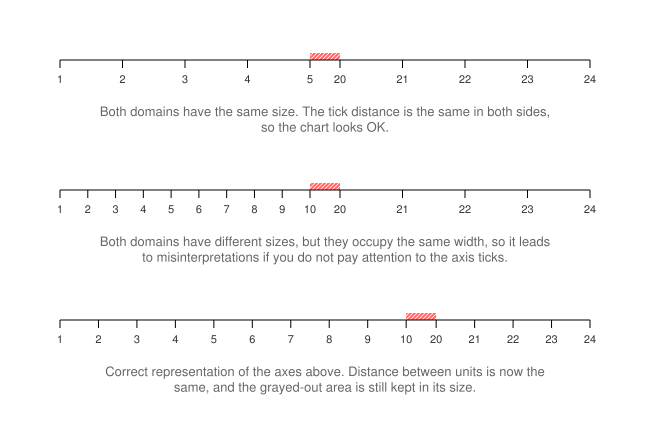2. If you want to highlight two events with very different sizes, you have to keep the domain size of both charts equivalent to the wider one (see above), which can make the smaller chart too compressed to be easily understood.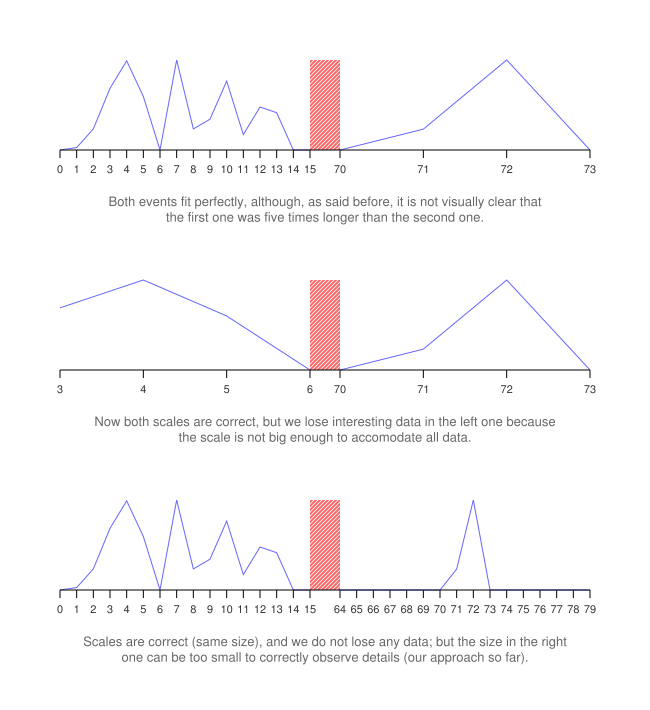3. This approach is hard to scale to show multiple broken axes, especially if the resulting pieces have different, arbitrary sizes.

### Creating a D3-compatible linear scale

Currently, most of our charting infrastructure is based on D3.js, a JavaScript library for manipulating documents based on data. In D3, you define ways of transforming points of your data (what D3 calls “the domain”) to pixels on the screen (what D3 calls “the range”) by using scales. D3 offers many types of scales, but a broken axis is usually shown in a linear scale.

Our goal was to create a D3-compatible linear scale, where you can strip out parts of the domain; that will still work when used instead of a standard linear scale.

Each domain interval to be plotted in the chart is called D, and we can have n of them, running from D0 to Dn-1. Each domain interval has a start (s) and an end (e), used in the form [s, e], which we define as Dsx and Dex.

Between each interval we will have a zone, representing the grayed-out area used for indicating the gap. The length of the gap is z, which is defined in range units, not in domain units, since we do not want it to grow or shrink depending on the size of the chart. In other words, z—which is always the same size—represents the width in pixels of the grayed-out zone and has nothing to do with the unit size of the domain.If we stretch the chart, then D0, D1, and D2 will double in size, but since z is defined in pixels, it has to remain the same size. This is the expected result:In order to transform from the domain to the range, which is the goal of the scale, we will convert this discontinuous set of intervals into a single, continuous interval that will span from Rs to Re (the starting value of the range to the ending value).

### The math of scales and ranges

So the problem looks like this: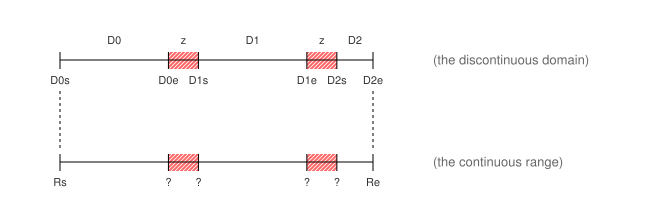The goal is to convert D intervals into R intervals so that later, when a point of the domain needs to be transformed into a point of the range, we just look up which D interval it is in, and do a linear interpolation.

To achieve this we need to compute the ratio between the domain and the range: how many units of the domain equal a unit in the range. In other words, how many pixels does a unit from the domain extend?

To do that we have to divide the width of the domain by the width of the range. We will call them Wd and Wr:

• Wd is equivalent to the sum of the sizes of all the domain intervals:• Wr is equivalent to the size of the range, minus the width of the grayed-out areas we have to draw. We remove them because their size is fixed and they have nothing to do with the domain. With n domain intervals, there are n-1 grayed areas, each of them with a z length: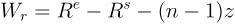Once we have the ratio, converting each domain interval (D) into a range interval (R) takes three steps:

1. Adding up all the sizes of the previous intervals (e.g., for R2 you would add D0 and D1), and multiplying them by the ratio (Wr/Wd).
2. Adding gaps (of z size) as needed (e.g., for R2 two gaps are needed: the one between D0 and D1, and the one between D1 and D2).
3. Adding the origin of everything, which is Rs.

Mathematically:andOnce we have all of these R intervals computed, to transform an arbitrary point d, we just have to look for the Dn where d ∈ Dn, then linearly interpolate this point to get it into the range (r):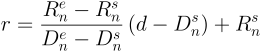We can also prove that Rs= Rs and that Ren-1 = Re; that is, the starting limit of the first range is the starting of the original range, and the ending limit of the last range is the ending of the original range.

### Conclusion

With this approach, we were able to build a D3 scale that works the exact same way other scales work, so we can plug it into our existing charting ecosystem.

We also improved the versatility of broken axes by being able to break them multiple times with arbitrary sizes, without worrying about creating a potentially misleading visual representation. This means other engineers don’t have to worry about complex equations to compute the sizes of various charts or the complex HTML they need to be properly displayed.

Our solution has other benefits. It enables the possibility of creating other broken axes, like logarithmic or exponential axes, for example, by performing a logarithm or exponentiation on the given point before passing it to the scale. This could be combined with standard D3 logarithmic and exponential scales so that we get all the existing power from D3 (e.g., meaningful ticks, nice boundaries, and so on). Give it a try and see if you can come up with your own uses for the technique!